office (412) 967-9367
email: ftsweb@gmail.com
toll-free 1 (800) 214-3480

3.3 Spot Rates and the Yield to Maturity

 I

The difference between spot rates and yields occurs with fixed-income securities that have intermediate cash flows, such as coupon bonds, when the spot rates for all maturities are not the same.

To illustrate the difference between spot rates and yields, let’s look at the problem of pricing a bond relative to the observed spot rates for different maturities. The price of the bond with coupon C, face value F, and maturity T, iswhere rt is the spot interest rate for maturity t.

Alternatively, given the observed market price, P, these spot rates can be replaced by the yield to maturity. This is the interest rate, (y for "yield") that solves:As you can see, the yield replaces all the different spot interest rates with a single interest rate.

Now, let P’ be the price of a fixed-income security that has the same maturity but different cash flows to the bond whose price is P: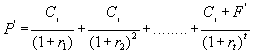and whose yield, y’, solves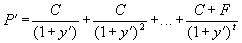If the cash flows are different, it is typically not the case that y = y’. Thus, two bonds with the same maturity can have different yields even if the same spot rates are used to calculate the value of each bond.

Example: Cash Flows, Spot Rates and Yield to Maturity

Assume that investors can borrow or lend at the respective spot rates for periods 1 to 3: 5%, 6%, and 7%. Now, consider two fixed-income securities that have the following pattern of cash inflows.

 Security Period 1 Period 2 Period 3 Security A \$10 \$10 \$110 Security B \$ 5 \$10 \$115

The arbitrage price of Security A is determined from the spot rates as follows: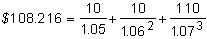Similarly, the arbitrage price of Security B is: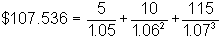The yield to maturity for Security A is the interest rate that equates the present value of the cash flows to the price \$108.216. You can verify that this rate equals 6.88%: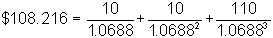Similarly, for Security B, you can verify that this rate equals 6.91%: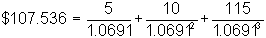In this example, even though the total cash inflows from the two securities are equal, Security A has a larger present value than Security B because the cash flows are received earlier (and therefore are discounted less) than in the case of Security B.

Reinvestment Rates and the Yield to Maturity

Frequently, the yield to maturity is used to measure the return from holding a bond. In fact, this is not an entirely accurate measure, since it assumes that you can reinvest intermediate coupons at the same yield. You earn the yield to maturity only if all cash flows are reinvested at the yield to maturity. If interest rates are not constant, however, you may not be able to reinvest the cash flows at that rate.

You will see more of this in Topic 3.7, Forward Interest Rates. Here, we explicitly discuss the rates at which you can reinvest cash flows.